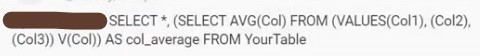# T-SQL: how to average the sum of columns in a new column with a query for the whole table?

Hello everyone and first of all congrats for this amazing community around (MS) SQL (Server)I am newbie to T-SQL intending to dive deep into the DB stuff, however, I am at the start of my journey with the following question. Imagine a table where we have the student id and 3 other entities which are, say, school courses' marks (in maths, biology, geography) stored as INT.

What is asked is to find the average marks for each student comprised as the total of the marks divided by their count.

How should the query look like to achieve this, since the AVG function is an aggregate one that is retrieving the average column-wise but not multiple column-wise. Is there an elegant way to achieve this by a query?

Could you please elaborate the below query or modify it so that it works? Is there an elegant way to make this using AVG and COUNT() only without the trivial sum/count?I found a solution that works but I am wondering if this could also work with usage of AVG() of SUM() and then division of the result by COUNT() so that we can retrieve the average mark of each student in a separate column.

`````````
``````
``````USE [Students]
GO

SET ANSI_NULLS ON
GO

SET QUOTED_IDENTIFIER ON
GO

CREATE TABLE [dbo].[Students](
[StudentID] [int] NOT NULL,
[StudentName] [nchar](100) NOT NULL,
[StudentSurname] [nchar](100) NOT NULL,
[Biology] [decimal](3, 2) NOT NULL,
[Maths] [decimal](3, 2) NOT NULL,
[Geography] [decimal](3, 2) NOT NULL,
CONSTRAINT [PK_Students] PRIMARY KEY CLUSTERED
(
[StudentID] ASC
)WITH (PAD_INDEX = OFF, STATISTICS_NORECOMPUTE = OFF, IGNORE_DUP_KEY = OFF, ALLOW_ROW_LOCKS = ON, ALLOW_PAGE_LOCKS = ON, OPTIMIZE_FOR_SEQUENTIAL_KEY = OFF) ON [PRIMARY]
) ON [PRIMARY]
GO

GO
INSERT [dbo].[Students] ([StudentID], [StudentName], [StudentSurname], [Biology], [Maths], [Geography]) VALUES (1, N'Mike                                                                                                ', N'Manson                                                                                              ', CAST(2.00 AS Decimal(3, 2)), CAST(2.00 AS Decimal(3, 2)), CAST(1.00 AS Decimal(3, 2)))
INSERT [dbo].[Students] ([StudentID], [StudentName], [StudentSurname], [Biology], [Maths], [Geography]) VALUES (2, N'Timo                                                                                                ', N'Torn                                                                                                ', CAST(1.00 AS Decimal(3, 2)), CAST(2.00 AS Decimal(3, 2)), CAST(4.00 AS Decimal(3, 2)))
INSERT [dbo].[Students] ([StudentID], [StudentName], [StudentSurname], [Biology], [Maths], [Geography]) VALUES (3, N'Jeffrey                                                                                             ', N'Jones                                                                                               ', CAST(1.00 AS Decimal(3, 2)), CAST(3.00 AS Decimal(3, 2)), CAST(4.00 AS Decimal(3, 2)))
GO

SELECT * FROM Students;

SELECT
(SELECT Biology + Maths + Geography)/3 AS Mark_average
FROM Students;
``````

The output ot the above trivial solution is:

``````Mark_average
1.666666
2.333333
2.666666
``````

Welcome

1st off do you want to talk about table design?

What if this table design was live in production and then suddenly the school now offers 10 more new courses, how would you handle that?

1 Like

Hello, thanks.

I know the table is not 3NF-compliant, I have created it ad-hoc just for the sake of the example only...1 Like
``````SELECT s.*, ca1.*
FROM Students s
CROSS APPLY (
SELECT SUM(subject) / COUNT(subject) AS Mark_average
FROM (
VALUES(Biology), (Maths), (Geography)
) AS subjects(subject)
) AS ca1``````
1 Like

Works like a charm, thanks much for the assistance.

I will have to research now about the cross apply, pivoting etc...